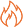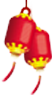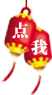# 全面解析一维数组

1.一维数组声明方式

2.一维数组中元素的表示方法

package com.shxt.demo01;

public class ArrayDemo01 {

public static void main(String[] args) {

int[] score = null; //声明数组,但是为开辟内存空间

score = new int; //为数组开辟"堆内存"空间

System.out.println("score="+score); //分别输出数组的每个元素

System.out.println("score="+score); //分别输出数组的每个元素

System.out.println("score="+score); //分别输出数组的每个元素

// 使用循环依次输出数组中的全部内容

for (int i = 0; i < 3; i++) {

System.out.println("score["+i+"]="+score[i]);

}

}

}

java.lang.ArrayIndexOutOfBoundsException:3

package com.shxt.demo01;

public class ArrayDemo02 {

public static void main(String[] args) {

int[] score = null; //声明数组,但是为开辟内存空间

score = new int; //为数组开辟"堆内存"空间

for (int i = 0; i < 5; i++) {

score[i] = i*2+1;

}

for (int i = 0; i < 5; i++) {

System.out.println("score["+i+"]="+score[i]);

}

}

}

package com.shxt.demo01;

public class ArrayDemo03 {

public static void main(String[] args) {

int[] score = new int;

System.out.println("数组长度为:"+score.length);

}

}

3. 一维数组的初始化方式

package com.shxt.demo01;

public class ArrayDemo04 {

public static void main(String[] args) {

int[] score = null; //声明数组,但是为开辟内存空间

score = new int; //为数组开辟"堆内存"空间

score = 100;

score = 200;

score = 300;

}

}

package com.shxt.demo01;

public class ArrayDemo04 {

public static void main(String[] args) {

int[] score = {10,20,30,40,50};

for (int i = 0; i < score.length; i++) {

System.out.println("score["+i+"]="+score[i]);

}

}

}

4一维数组一定要初始化吗

package com.shxt.demo01;

public class ArrayDemo08 {

public static void main(String[] args) {

// 静态初始化:定义并且初始化nums数组

int nums[] = {13,34,57,100};

// 定义一个scores数组变量

int[] scores;

//让scores数组执行nums所引用的数组

scores = nums ;

// 对scores进行变量

System.out.print("scores数组数据:");

for (int i = 0; i < scores.length; i++) {

System.out.print(scores[i]+"\t");

}

// 将scores数组中第3个元素赋值为200

scores = 200;

// 访问 nums 数组的第3个元素,将看到输出值为200

System.out.println("\nnums 数组的第3个元素,将看到输出值为:"+nums);

}

}

package com.shxt.demo01;

public class ArrayDemo09 {

public static void main(String[] args) {

// 静态初始化:定义并且初始化nums数组

int nums[] = {13,34,57,100};

// 引用传递, int[] temp = nums

print(nums);

}

public static void print(int[] temp){

for (int i = 0; i < temp.length; i++) {

System.out.print(temp[i]+"\t");

}

}

}

package com.shxt.demo01;

public class ArrayDemo10 {

public static void main(String[] args) {

// 静态初始化:定义并且初始化nums数组

int nums[] = {1,2,3,4,5};

// 引用传递, int[] temp = nums

inc(nums); //扩容操作

print(nums); //打印内容

}

public static void inc(int[] temp){

for (int i = 0; i < temp.length; i++) {

temp[i]*=2;

}

}

public static void print(int[] temp){

for (int i = 0; i < temp.length; i++) {

System.out.print(temp[i]+"\t");

}

}

}HOT资讯 >

• 姓名
• 手机
• 性别
• 意向课程